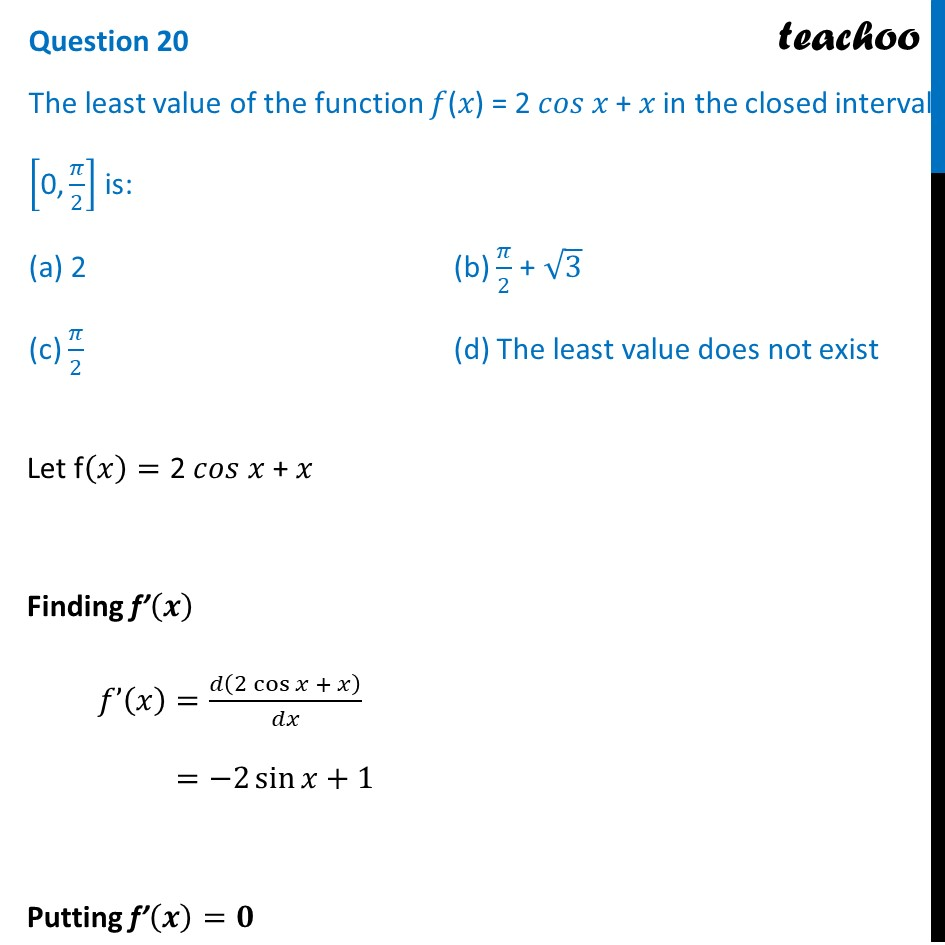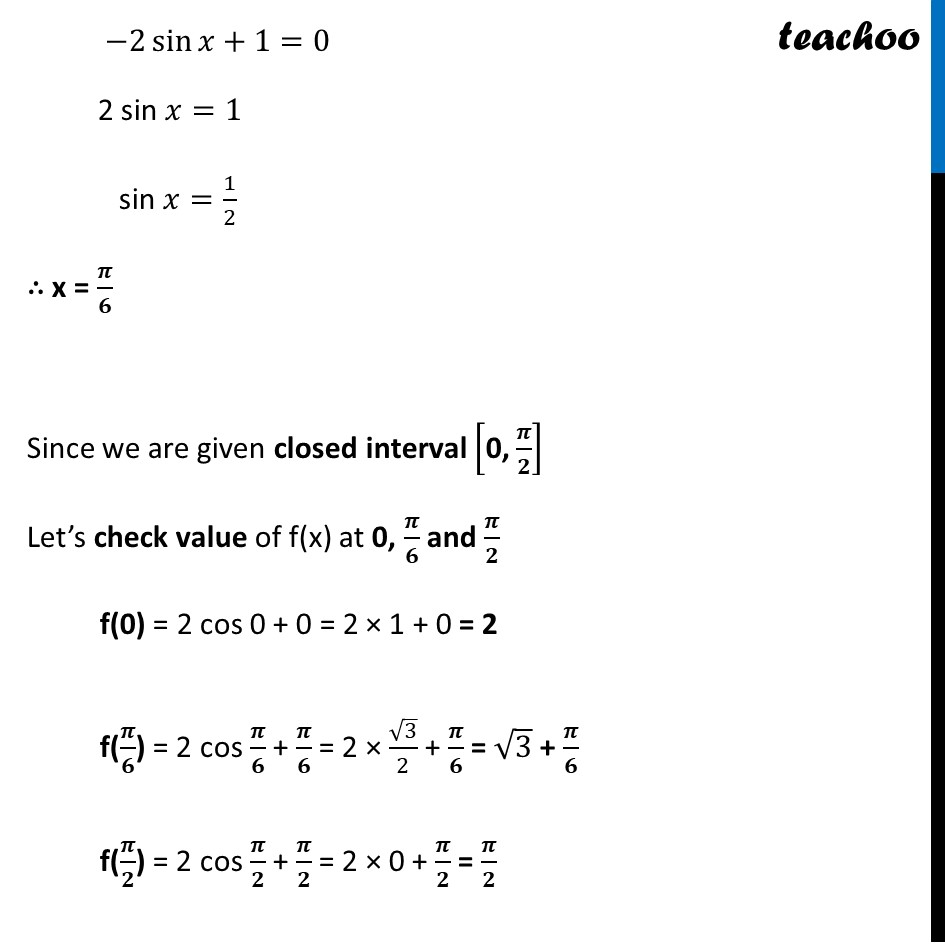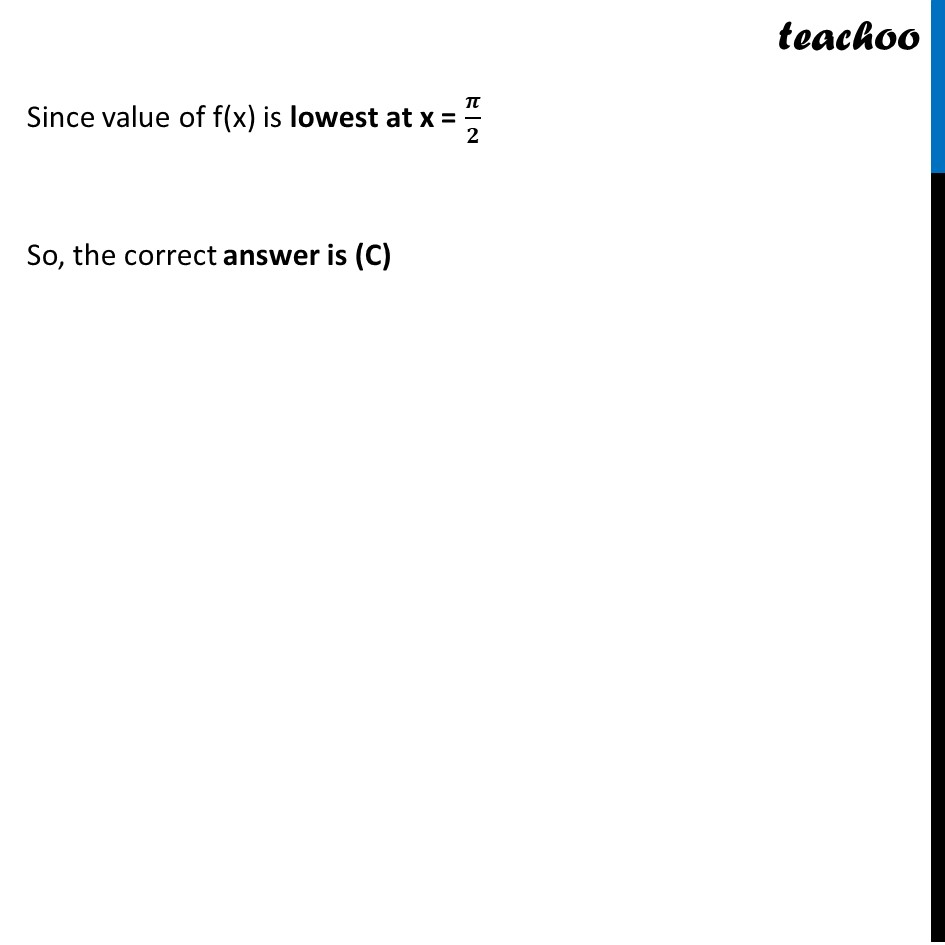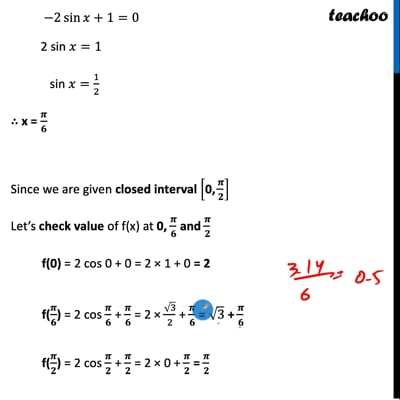CBSE Class 12 Sample Paper for 2022 Boards (MCQ Based - for Term 1)

Class 12
Solutions of Sample Papers and Past Year Papers - for Class 12 Boards

## (a) 2       (b) π/2 + √3 (c) π/2    (d) The least value does not existThis video is only available for Teachoo black users

Introducing your new favourite teacher - Teachoo Black, at only ₹83 per month

### Transcript

Question 20 The least value of the function 𝑓(𝑥) = 2 𝑐𝑜𝑠 𝑥 + 𝑥 in the closed interval ["0," 𝜋/2] is: (a) 2 (b) 𝜋/2 + √3 (c) 𝜋/2 (d) The least value does not exist Let f(𝑥)="2 𝑐𝑜𝑠 𝑥 + 𝑥" Finding f’(𝒙) 𝑓’(𝑥)=𝑑(2 cos⁡𝑥 + 𝑥)/𝑑𝑥 =−2 sin⁡𝑥+1 Putting f’(𝒙)=𝟎 −2 sin⁡𝑥+1=0 2 sin 𝑥=1 sin 𝑥=1/2 ∴ x = 𝝅/𝟔 Since we are given closed interval ["0," 𝝅/𝟐] Let’s check value of f(x) at 0, 𝝅/𝟔 and 𝝅/𝟐 f(0) = 2 cos 0 + 0 = 2 × 1 + 0 = 2 f(𝝅/𝟔) = 2 cos 𝝅/𝟔 + 𝝅/𝟔 = 2 × √3/2 + 𝝅/𝟔 = √3 + 𝝅/𝟔 f(𝝅/𝟐) = 2 cos 𝝅/𝟐 + 𝝅/𝟐 = 2 × 0 + 𝝅/𝟐 = 𝝅/𝟐 Since value of f(x) is lowest at x = 𝝅/𝟐 So, the correct answer is (C)